﻿ ﻿Lines and Angles - ESSENTIAL GEOMETRY SKILLS - SAT Test Prep

## CHAPTER 10ESSENTIAL GEOMETRY SKILLS

1. Lines and Angles

2. Triangles

3. The Pythagorean Theorem

4. Coordinate Geometry

5. Areas and Perimeters

6. Similar Figures

7. Volumes and 3-D Geometry

8. Circles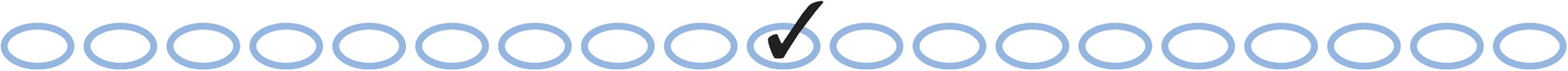### Lesson 1: Lines and Angles

When Two Lines Cross

When two lines cross, four angles are formed. “Vertical” angles are equal, and look like this:Don”t be fooled by diagrams that look like vertical angles, but aren”t. Vertical angles are formed by two and only two crossed lines:When two pairs of vertical angles are formed, four pairs of adjacent angles (side-by-side) are also formed. Adjacent angles add up to 180°: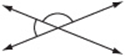When a Line Crosses Parallel Lines

Imagine taking two crossed lines, making a “copy” of them, and sliding the copy down one of the lines so that together they look like this:This produces a pair of parallel lines crossed by a third line.

When two parallel lines are crossed by another line, all acute angles are equal, and all obtuse angles are equal. Also, every acute angle is supplementary to every obtuse angle (that is, they add upto 180°).

To show that two lines are parallel, use the arrow marks “>” like those in the figure in the previous column. To show that two angles are equal, use the arc marks “) ” like those in the figure in the previous column.

Don”t be fooled by diagrams that only look as if they have two parallel lines crossed by another line. Don”t assume that two lines are parallel just because they look parallel. It must be given that they are parallel.

To help yourself to see the relationships between angles in parallel line systems, you might try looking for these special “letters”:

Angles that make Z”s are equal:Angles that make C”s or U”s are supplementary (they have a sum of 180°):Angles that make F”s are equal:Concept Review 1: Lines and Anglesl1 and l2 are lines and r is a ray.

Questions 1 and 2 refer to the diagram above.

1. List all of the different pairs of angles that are congruent (equal).

2. List all of the different sets of angles that have a sum of 180°.

Mark the figure to show the following information: AD || HN, AI || BM, and HD || JL. Then list the angles in the figure that have the given characteristic:3. One angle equal to ∠10

4. Two angles supplementary to ∠6

5. Two angles supplementary to ∠9

6. One angle equal to ∠15

7. Three angles supplementary to ∠13

State whether each of the following pairs is supplementary (has a sum of 180°), equal, or neither.

8. ∠13 and ∠7

9. ∠13 and ∠6

10. ∠2 and ∠9

11. ∠14 and ∠9

12. ∠7 and ∠3

13. ∠1 and ∠2

14. ∠6 and ∠7

SAT Practice 1: Lines and Angles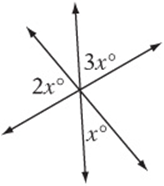Note: Figure not drawn to scale.

1. The figure above shows the intersection of three lines. x =

(A) 16

(B) 20

(C) 30

(D) 60

(E) 902. The figure above shows a parallelogram with one side extended. If, then y =

(A) 40

(B) 60

(C) 80

(D) 110

(E) 120Note: Figure not drawn to scale.

3. In the figure above, if ℓ1||ℓ2, then(A) 130

(B) 270

(C) 280

(D) 290

(E) 3104. In the figure above, if l1|| l2, then what is the value of n in terms of m?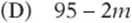5. In the figure above, if l1|| l2, then x =

(A) 43

(B) 69

(C) 79

(D) 101

(E) 1116. In the figure above, ifandbisects ∠HFG, what is the measure of ∠FJH?

(A) 14

(B) 38

(C) 40

(D) 56

(E) 76Note: Figure not drawn to scale.

7. In the figure above, if ℓ1||ℓ2 and ℓ2||ℓ3, then a =

(A) 50

(B) 55

(C) 60

(D) 65

(E) 70Note: Figure not drawn to scale.

8. In the diagram above, if ℓ1||ℓ2, then x =

(A) 65

(B) 60

(C) 50

(D) 45

(E) 40

Answer Key 1: Lines and Angles

Concept Review 1

1. Only c and e are congruent.,,,3. ∠5

4. ∠7 and ∠13

5. ∠12 and ∠14

6. ∠4

7. ∠11, ∠8, and ∠6

8. equal

9. supplementary

10. neither

11. supplementary

12. neither

13. supplementary

14. supplementary

SAT Practice 1

1. C Draw in the three angles that are “vertical,” and therefore congruent, to the angles that are shown. Then choose any three adjacent angles, and notice that they form a straight angle. Therefore,. Soand.

2. B The opposite angles in a parallelogram must be equal, and any two “consecutive angles” as you move around the figure must be supplementary. (Notice that consecutive angles form C”s or U”s. If you”re not sure why this theorem is true, sketch a few sample parallelograms and work out the angles.) The angle opposite the must also measure , and when this is added to the three angles, they form a straight angle. Therefore,and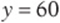.

3. E In the triangle, the angles must have a sum of 180°. (See the next lesson for a simple proof.) Therefore, the other two angles in the triangle must have a sum of 50°. Pick values for these two angles that add up to 50°, and write them in. It doesn”t matter how you do it: 25° and 25°, 20° and 30°, 40° and 10°, as long as they add up to 50°. You can then find the values of a and b by noticing that they form straight angles with the interior angles. So if the interior angles are 25° and 25°, then a and b must both be 155.

4. C Notice that the angle has a “corresponding” angle below that has the same measure. (Notice that they form an F.) Then.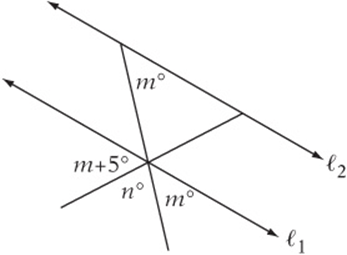5. C Draw an extra line through the vertex of the angle that is parallel to the other two. Notice that this forms two “Z” pairs. Therefore,.6. B There are many relationships here to take advantage of. Notice that a ∠HFG and the 76° angle form a “Z,” so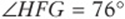. Remember that “bisect” means to divide into two equal parts, so. Then notice that. ∠JFG and∠FJH form a “Z,” so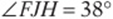.7. A Consider the triangle with the angle. The other two angles in the triangle can be found from the given angles. Notice the “Z” that contains the two 80° angles and the “U” that contains the 130° and 50° angles. Therefore,, so.8. E Draw two more parallel lines and work with the Z”s. Your figure should look like the one above.

﻿